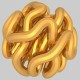## 1. E ≤ 3V

Let G be a simple planar graph with V vertices and E edges. It follows from Euler’s theorem that

## E ≤ 3V

In fact, we have (when V is at least 3,) that E 3V – 6.

To see this,  denote by F the number of regions or faces determined by G (in other words, the number of connected components in the complement of the embedded graph). Euler’s theorem asserts that

E – V + F = 2

## V – E + F = 2

and now note that every face must have at least three edges and every edge is contained in two faces and therefore$2E \ge 3F$, so 6=3V – 3E + 3F ≤ 3V – 3E +2E.

## 2. F ≤ 4E

Now let K be a two-dimensional simplicial complex and suppose that K can be embedded in$R^4$. Denote by E the number of edges of K and by F the number of 2-faces of K.

Here is a really great conjecture:

Conjecture:

## F ≤ 4E

A weaker version which is also widely open and very interesting is:

For some absolute constant C,

## F ≤ C E

Remarks: The conjecture extends to higher dimensions. If K is an r-dimensional simplicial complex that can be embedded into$R^{2r}$ then the conjecture is that$f_r(K) \le C_rf_{r-1}(K),$

Where$C_r$ is a constant depending on r.  Here$f_i(K)$ is the number of i-dimensional faces of K. A stronger statement is that$C_r= r+2$. The conjecture also extends to polyhedral complexes and more general form of complexes. In the conjecture ’embed’ refers to a topological embedding.

This entry was posted in Combinatorics, Convex polytopes, Geometry, Open problems and tagged . Bookmark the permalink.

### 14 Responses to F ≤ 4E

1.Terence Tao says:

Some minor nitpicks: Euler’s formula is strictly speaking only valid for connected graphs, but the bound$E \leq 3V$ for connected graphs implies the same bound for general graphs (one needs the non-strict inequality to deal with the empty graph though).

Also, the bound$E \leq 3V-6$ can fail for really small graphs because they can have faces with fewer than three edges, or edges in which both sides belong to the same face. (Someone pointed this out to me when I tried to use this stronger bound to prove the crossing number inequality.)

•Gil Kalai says:

Dear Terry, thanks! I replaced now the strict inequalities by weak ones which presented a typographical challenge. (Also it is sometimes useful to think about 3V-6 as really representing${{V} \choose {2}}-{{V-3} \choose {2}}$ and then it works for all values of V.)

2.Danny Calegari says:

Hi Gil – do you ask to embed your simplicial complex K in R^4 topologically/smoothly, or perhaps linearly (on each simplex)?

•Danny Calegari says:

Oops – didn’t read the last line (topological embedding); just to be sure – disks can be embedded topologically in R^4 in pretty complicated ways (locally) – do you mean locally flat embeddings?

•Gil Kalai says:

Dear Danny, I mean indeed topological embedding.

3.J_Dime says:

Dear Prof. Kalai,

I apologize in advance for what may be a stupid question,
but why is 4 the conjectured number?

It may be my impaired 4-D vision, but I couldn’t think of anything (non-empty, of course) even approaching F=4E.

Could you give a critical example or two, having a high E-to-F ratio?

Thank you very much!

•Gil Kalai says:

Dear J_Dime, it can be useful to think about the 2-skeleton of triangulations of 4-spheres, and if you take the triangulation described by the cyclic 5-polytope this allows you to approach 4. you can think for this case about n vertices 1,2,…,n the edgers are all pairs of vertices and the 2-faces are triangles of the form 1 i j or i j n or i i+1 j or i j j+1 where i<j (and in the third case also i+1<j).

4.Michal says:

Dear Gil,

1. What about the special case when K is the 2-skeleton of a 4-sphere (as in your comment above)? Is that also not known?

2. What about a weaker conjecture that F<C*V^2 ? Is that also open?

•Gil Kalai says:

Dear Michal, The special case when K is the 2-skeleton of a 4 sphere is also wide open and very interesting. If the 4-sphere is polytopal or even if K can be embedded as a subcomplex of the boundary complex of a simplicial 5-polytope then it is known.

2) Hmm, I thought that this is known but now that I think about it I am not sure.

5.Tamal Dey says:

It is encouraging to see that this question has been asked in this forum, one that I asked in my early years of research (1992-1993) and couldn’t find an answer. Last year I raised the question again in a Oberwolfach meeting. Please see the document http://www.mfo.de/document/1218/OWR_2012_24.pdf (Open problem 14, page 1481) which I co-wrote with Uli Wagner on what little known on the topic.

•Gil Kalai says:

Dear Tamal, thanks for the comment.